Automation, Control and Intelligent Systems
Volume 3, Issue 3, June 2015, Pages: 36-38

Robustness and Stability Margins of Linear Quadratic Regulators

Aref Shahmansoorian, Sahar Jamebozorg

EE Department, Imam Khomeini International University, Qazvin, Iran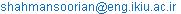(A. Shahmansoorian)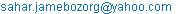(S. Jamebozorg)

Aref Shahmansoorian, Sahar Jamebozorg. Robustness and Stability Margins of Linear Quadratic Regulators. Automation, Control and Intelligent Systems. Vol. 3, No. 3, 2015, pp. 36-38. doi: 10.11648/j.acis.20150303.12

Abstract: In this paper, It is showed that however we can mention the guaranteed gain margin of -6 to +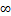and also phase margin of -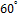to +for single input systems as the well-known robustness properties of linear quadratic regulators (LQR). But determining the robustness of closed-loop system from the range of gain and phase margins is not corrected. By an example, this matter is explained.

Keywords: Linear Quadratic Regulators, Robustness, Gain Margins, Phase Margins

Contents

1. Introduction

In classical frequency domain techniques for single-input single-output (SISO) control system design, the robustness issue is handled . These techniques employ various graphical means (e.g., Nyquist, inverse Nyquist, Bode, Nichols plots) of displaying the system model in terms of its frequency response. In these plots, it is automatic to determine the minimum change in the model frequency response that leads to instability.

The margins are defined in . Here the nominal feedback system is assumed stable. The positive phase margin is the smallest value of Φ greater than 0 such that the system of Fig. 1 with L(j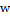) =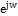is unstable. The issue of robustness of linear-quadratic regulators (LQR) were very attractive for many years. Phase margin is defined in a similar style. The upward gain margin is the smallest value of L(s) = constant > 1 (usually L(s) = +1 ) for which the system is unstable, and the downward gain margin is similarly defined. The notions of gain and phase margins have gained such wide-spreading acceptance that they are incorporated in to the specifications for a control system design .

Each LQR for a single-input plant possesses a guaranteed gain margin of -6 to +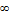and phase margin of -to +, in all channels [3,4,5]. It is demonstrated via an example that in spite of its impressive margins the full state linear quadratic optimal regulator may suffer from robustness problems where small changes in the parameters of the system may lead to fast unstable closed-loop modes. The gain and phase margins of the optimal regulator do not guarantee good robustness to plant parameter variation [6, 7,8].

In this paper, the same linear quadratic state feedback optimal regulator problem is considered and it is shown the conclusion is not correct and cannot opine about robustness of the plant at this way.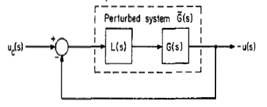Fig. 1. Feedback system with multiplicative representation of uncertainty in G(s) .

2. Example

We consider the same linear quadratic state feedback optimal regulator problem . For the single input, single output linear time-invariant continuous system S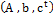given by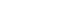(1)

Where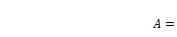(2)

A state feedback control should be found that minimizes the following index of performance: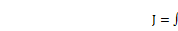(3)

It is well known that the optimal control law is given by: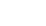(4)

Where the optimal gain vector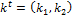satisfies the following spectral factorization equation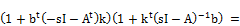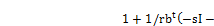(5)

The return difference of the optimal regulator is readily given by: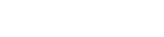(6)

Where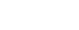(7)

From the latter the optimal gain vector is found to be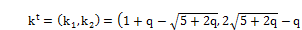(8)

Perturbing b by small variationand considering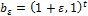examine the zeros of the new return difference. We obtain that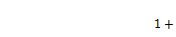(9)

Where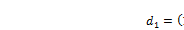(10)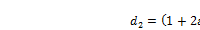(11)

In  is used Fig. 2 for determining the instability region of the system, first it is created a question in each reader's mind "why is the diagram based on". Whiles Fig. 3 shows the corrected diagram. As can be seen in Fig. 2 if 1/r is selected great so the system will be unstable. In fact with selection of great 1/r and smallwe can determine the instability region but pay attention that great 1/r means small r in Fig. 3 and however r goes smaller value the effect of optimal control law becomes lower in index of performance so that in very large 1/r (very small r) the index performance will be nearly the following form.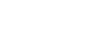(12)

In this case the amplitude of control policy can be very large so each small variation in parameters (each small) causes that the control idea becomes large and finally the plant goes to instability.

On the other hand, the optimal control law is not designed for contrasting against uncertainty in parameters. However by using the optimal control policy the system possesses the guaranteed stability margins, but stability margins are used for defining instability or stability of the plant not for the robustness. In other words gain and phase margins of the system show acceptable variations of gain or phase of the controller, while the closed loop system is stable.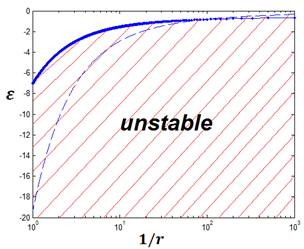Fig. 2. Instability region of the closed loop system .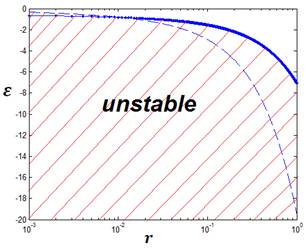Fig. 3. New instability region of the closed loop system.

Now we suppose b is changed fromto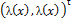, it means the linear system is converted to a nonlinear system, in this case if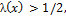the plant remains stable. In addition, if we consider that one of the components of b is zero, that is, b equals to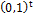or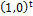, with Perturbing b by small variationin nonzero component for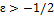the system will be stable.

3. Conclusion

In this paper it has been shown that cannot use the stability margins for diagnosis of the robustness of the plant because gain and phase margins are applied for recognition instability or stability of the plant not for robustness. In this work we illustrate that high gain margin of the system never insure being robustness against uncertainties also the gain margin and robustness of the plant versus the each variation in the parameters are the separate concepts. Moreover it can be found the optimal control law of the linear quadratic regulators is robust against the special range of variations in input gain factor. So linear quadratic multivariable designs have the property of gain margin of -6 to +and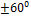phase margin for each control channel that cannot use from this feature for denotation of robustness of the plant.

References

1. Horowitz, Isaac M. Synthesis of feedback systems. Elsevier, 2013.
2. N. A. Lehtomaki, N. R. Sandell, Jr, and M. Athans, "Robustness results in linear-quadratic Gaussian based multivariable control designs", IEEE Trans. Automatic Control, Vol. AC-26. pp. 75-93, Feb. 1981.
3. B.D.O. Anderson, "The inverse problem of optimal control", Stanford Electronics Laboratories, Stanford, CA, Tech. Rep. SEL-66-038 (T. R. no.6560-3), Apr. 1966.
4. B. D. O. Anderson and J. B. Moore, Linear Optimal Control. Englewood Cliffs, NJ: Prentice-Hall, 1971.
5. R. E. Kalman, "When is a linear control system optimal?", Trans. ASME Ser. D: J. Basic Engineering, Vol. 86, pp. 51-60, Mar. 1964.
6. E. Soroka, U. Shaked, "On the robustness of LQ regulators", IEEE Trans, Automatic Control, Vol. AC-29, no. 7, pp. 664-665, 1984.
7. J. C. Doyle, ‘Guaranteed margins for LQG regulators. "IEEE Truns. Auromur. Conrr., vol. AC-23. pp. 756-757. Aug. 1978.
8. A. Khaki-Sedigh, Analysis and design of Multivariable Control Systems, , K. N. Toosi University Press, 2011.
9. Anderson, Brian DO, and John B. Moore. Optimal control: linear quadratic methods. Courier Corporation, 2007.

 Contents 1. 2. 3.
Article ToolsAbstractPDF(194K)